# RBSE Maths Class 10 Chapter 12: Circle Important Questions and Solutions

RBSE Class 10 Maths Chapter 12 – Circle Important questions and solutions are given here. All these questions provided here are with stepwise solutions and are given as per the new syllabus and guidelines prescribed by the board. The RBSE Class 10 important questions and solutions available at BYJU’S will help the students to understand all the topics of class 10 better and score maximum marks.

Chapter 12 of RBSE Class 10 has four exercises with several concepts of the circle and related theorems. Different theorems are given, which prove the statements related to chords and their properties. Besides, all the important questions given here will help the students to understand the way questions occur in the examination and how to solve them correctly.

### RBSE Maths Chapter 12: Exercise 12.1 Textbook Important Questions and Solutions

Question 1: Fill in the blanks.

(i) Centre of a circle is situated in the ______ of the circle. (exterior / interior)

(ii) A point whose distance from the centre of the circle is greater than its radius lies in the ______ of the circle. (exterior / interior)

(iii) The longest chord of a circle is a ______ of the circle.

(iv) An arc is a ______ when its ends are then ends of a diameter of a circle.

(v) A circle divides the plane, on which it lies in ______ parts.

Solution:

(i) Centre of a circle is situated in the interior of the circle.

(ii) A point whose distance from the centre of the circle is greater than its radius lies in the exterior of the circle.

(iii) The longest chord of a circle is a diameter of the circle.

(iv) An arc is a semicircle when its ends are then ends of a diameter of a circle.

(v) A circle divides the plane, on which it lies in three parts.

(i) Line segment joining the centre to any point on the circle is a radius of the circle.

(ii) In a circle a number of equal chords are finite.

(iii) If a circle is divided into three equal arcs, then each arc is a major arc

(iv) A chord of a circle, which is twice as long as its radius, is a diameter of the circle.

(v) A circle is a plane figure.

(vi) In a plane the set of points is a constant distance from the fixed point on the same plane, it is diameter.

(vii) The chord which lies on the centre, it is known as radius.

Solution:

(i) True – Distance from a point on the circle to its centre is called radius.

(ii) False – Because there are an infinite number of points that exist on the circle. By joining any two we can get a set of equal infinite chords.

(iii) False – Since the circle is divided into three equal arcs. Thus, there will be no major arc in this case.

(iv) True – Diameter is the longest chord and its length is twice the radius.

(v) True – Circle is the locus of a point which moves in a plane at constant distance from a fixed point and hence, circle is a plane figure.

(vi) False – A circle is a collection of all points in a plane which are at a fixed distance from a fixed point.

(vii) False – If the chord passes through the center, then it is called diameter.

### RBSE Maths Chapter 12: Exercise 12.2 Textbook Important Questions and Solutions

Question 3: Write true or false and if possible, then give the reason for your answer.

(i) Chords AB and CD of a circle are 3 cm and 4 cm which make angles at the centre are 70° and 50° respectively.

(ii) The chords of a circle of length 10 cm and 8 cm. Which apart from the centre are 8 cm and 5 cm respectively.

(iii) Two chords AB and CD of a circle which are 4 cm distance from the centre then AB = CD.

(iv) Two congruent circle of centres O and O’ intersect each other at point A and B then ∠AOB = ∠AO’B.

(v) One circle can be drawn by three points which are in a line.

(vi) A circle of radius 4 cm can be drawn passing two points A and B, if AB = 8 cm.

Solution:

(i) False – Larger chord subtends larger angle as compared to the smaller chord.

(ii) False – Larger chord situated at a small distance from the centre of the circle.

(iii) True – Both chords are at equal distance from the centre.

(iv) True – Equal chords of congruent circles subtends equal angles at corresponding centres of circles.

(v) False – A circle which passes through two points cannot pass through the third point of the line.

(vi) True – AB is the diameter.

Question 4: If the radius of a circle is 13 cm and the length of its one chord is 10 cm, then find out the distance of this chord from the centre of the circle.

Solution:

Let AB be the chord and O be the centre of the circle.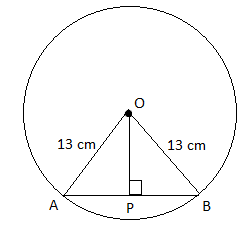AB = 10 cm

OA = OB = 13 cm

OP ⊥ AB.

We know that the perpendicular drawn from the center of the circle bisects the chord.

i.e. AP = PB = (1/2)AB = (1/2) × 10 = 5 cm

In right triangle OPB,

OB2 = OP2 + PB2

132 = OP2 + 52

⇒ OP2 = 169 – 25

⇒ OP2 = 144

⇒ OP = 12 cm

Hence, the distance of the chord from the centre of the circle is 12 cm.

Question 5: Two chords AB and CD of a circle whose lengths are 6 cm and 12 cm, respectively are parallel to each other and also situated on one side of the centre of the circle. If the distance between AB and CD is 3 cm, then find out the radius of the circle.

Solution:

Let O be the center, OA and OB be the radius of the circle, whose chords AB = 6 cm, CD = 12 cm.

Draw OP ⊥ AB which cuts the CD at Q.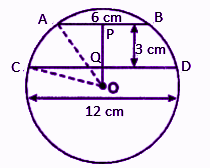AB || CD

OQ ⊥ CD

OP⊥ AB

AP = PB (perpendicular drawn from the center of the circle bisects the chord)

AP = PB = (1/2)AB = (1/2) × 6 = 3 cm

Similarly,

CQ = QD = (1/2)CD = (1/2) × 12 = 6 cm

Distance between the two chords AB and CD = MN = 3 cm

Let OQ = x

In right triangle OCQ,

OC2 = OQ2 + CQ2

OC2 = (x)2 + (6)2

OC2 = x2 + 36 ….(i)

In right triangle OAP,

OA2 = AM2 + OM2

⇒ OA2 = AM2 + (ON + MN)2

⇒ OA2 = AM2 + (x + 3)2

⇒ OA2 = (3)2 + (x + 3)2

⇒ OA2 = 9 + x2 + 9 + 6x …..(ii)

OA = OC (radius of the same circle)

⇒ OA2 = OC2 ….(iii)

From (i), (ii) and (iii),

x2 + 36 = 9 + x2 + 9 + 6x

⇒ 36 = 6x + 18

⇒ 6x = 36 – 18

⇒ 6x = 18

⇒ x = 18/6 = 3 cm

Substituting x – 3 cm in (i),

OC2 = (3)2 + 36

= 9 + 36

= 45

OC = √45 = 3√5 cm

Therefore, the radius of the circle is 3√5 cm.

Question 6: In the figure, AB and CD intersect each other at point E. Prove that: arc DA = arc CB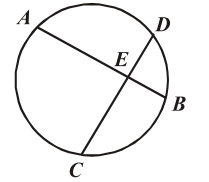Solution:

Given,

Two equal chords AB and CD intersecting each other at E.

Let O be the centre of the circle.

Join OA, OB, OC and OD.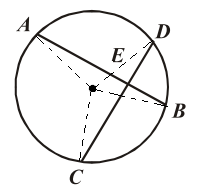We know that corresponding arcs of equal chords are equal also, equal arcs subtends equal angles at the center of the circle.

arc AB = arc CD

⇒ ∠AOB = ∠COD

Subtracting ∠BOD on both sides,

⇒ ∠AOB – ∠BOD = ∠COD – ∠BOD

⇒ ∠AOD = ∠COB

⇒ arc DA = arc CB

Hence proved.

Question 7: In the figure, AB and CD are two equal chords of a circle, O is the centre of the circle. If OM ⊥ AB and ON ⊥ CD, then prove that: ∠OMN = ∠ONM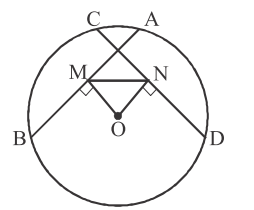Solution:

Given,

AB and CD are two equal chords of a circle, O is the centre of the circle.

OM ⊥ AB and ON ⊥ CD

We know that equal chords of a circle are equidistant from the center.

⇒ OM = ON

Thus, ΔOMN is an isosceles triangle.

In isosceles triangle angles opposite to equal sides are equal.

⇒ ∠OMN = ∠ONM

Hence proved.

Question 8: In the figure, O and O’ are the centres of circles. AB ∥ OO’, prove that: AB = 2OO’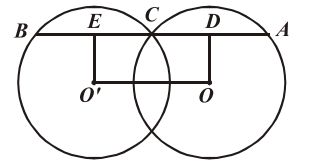Solution:

Given,

O and O’ are centre of two circles and AB || OO’ and OD ⊥ AB and O’E ⊥ AB.

AB || OO’ and ED || OO’ ….(i)

Thus, ED || OO’

Also, OD ⊥ AB, O’E ⊥ AB

OD = O’E

⇒ OO’ED is a parallelogram whose one angle is the right angle.

⇒ OO’ED is a rectangle so ED = OO’ ….(ii)

We know that the perpendicular drawn from the centre bisects the chord.

Thus, CD = AD, CE = BE

AB = BE + CE + CD + AD

= CE + CE + CD + CD

= 2CE + 2CD

= 2(CE + CD)

= 2ED

From (i) and (ii),

AB = 2OO’

Hence proved.

Question 9: AB and CD are two chords of a circle that AB = 10 cm and CD = 24 cm and AB ∥ CD. The distance between AB and CD is 17 cm. Calculate the radius of the circle.

Solution:

Let O be the center and r be the radius of the circle. OP ⊥ AB and OQ ⊥ AB.

Given,

AB and CD are two chords of a circle such that AB = 10 cm and CD = 24 cm and AB ∥ CD.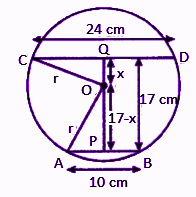AB || CD thus, P, O, Q will lie on the same line.

AP = BP = (1/2)AB = (1/2) × 10 = 5 cm

CQ = QD = (1/2) CD = (1/2) × 24 = 12 cm

PQ = 17 cm

Let OQ = x cm and OP = (17 – x) cm

In right triangle OPA,

By Pythagoras theorem,

OA2 = OP2 + AP2

r2 = (17 – x)2 + (5)2

r2 = (17 – x)2 + 25 …..(i)

In right triangle OQC,

OC2 = OQ2 + CQ2

r2 = x2 + (12)2

r2 = x2 + 144 ….(ii)

From (i) and (ii),

(17 – x)2 + 25 = x2 + 144

289 + x2 – 34x + 25 – x2 – 144 = 0

-34x + 170 = 0

⇒ 34x = 170

⇒ x = 170/34

⇒ x = 5 cm

Substituting x = 5 cm in (ii),

r2 = (5)2 + 144

r2 = 25 + 144

r2 = 169

r = √169 = 13

Therefore, the radius of the circle is 13 cm.

Question 10: Vertices of a cyclic quadrilateral are situated in such a way that AB = CD, then prove that AC = BD.

Solution:

Given,

AB = CD

Vertices A, B, C and D of a quadrilateral ABCD lie on the circle.

Join AC and BD.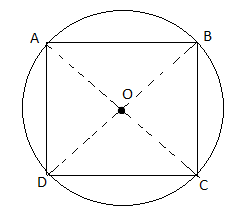AB = CD (given)

⇒ arc AB = arc CD

⇒ arc AB + arc BC = arc CD + arc BC

⇒ arc(AB + BC) = arc(CD + BC)

⇒ arc ABC = arc BCD

⇒ arc AC = arc BD

⇒ AC = BD

Hence proved.

Question 11: If two equal chords of a circle intersect each other, then prove their successive parts will be equal to their corresponding parts.

Solution:

Let O be the centre and AB, CD be the two equal chords intersecting at P.

Draw QM ⊥ AB and ON ⊥ CD and join OP.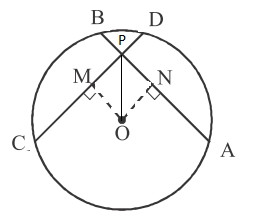OM ⊥ AB and ON ⊥ CD

⇒ OM = ON ….(i) (equal chords are equidistant from the centre)

In right ∆OMP and ∆ONP,

OM = ON [From (i)]

∠OMP = ∠ONP (each 90°)

OP = OP (common)

∴ ΔOMP = ΔONP

⇒ MP = PN ….(ii)

AB = CD

⇒ (1/2)AB = (1/2)CD

AM = CN ….(iii)

MP + AM = PN + CN

AP = PC ….(iv)

AB = CD ….(v)(given)

Subtracting (iv) from (v),

AB – AP = CD – CF

PB = PD

Hence proved.

Question 12: Prove that the bisector of two parallel chords passes through the centre of the circle.

Solution:

Let AB and CD be the two parallel chords of a circle with centre O and radius r.

Let P and Q be the midpoints of chords AB and CD respectively.

Join OP and OQ.

Draw line XOY ∥ AB.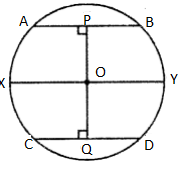OP bisects AB.

OP ⊥ AB

⇒ ∠BPO = 90°

But XY || AB then

∠BPO + ∠YOP = 180°

90° + ∠YOP = 180°

⇒ ∠YOP = 180°- 90°

⇒ ∠YOP = 90°

Q is the midpoint of the chord CD.

Thus, OQ ⊥ CD

⇒ ∠OQD = 90°

CD || XY

∠OQD + ∠YOQ = 180°

90° + ∠YOQ = 180°

∠YOQ = 180° – 90°

∠YOQ = 180° – 90°

∠YOQ = 90° ….(ii)

From (i) and (ii),

∠YOP + ∠YOQ = 90° + 90° = 180°

∠YOP + ∠YOQ = 180° (linear pair)

Therefore, POQ is a straight line.

### RBSE Maths Chapter 12: Exercise 12.3 Textbook Important Questions and Solutions

Question 13: In the figure, if ∠ABC = 45°, then prove that: OA ⊥ OC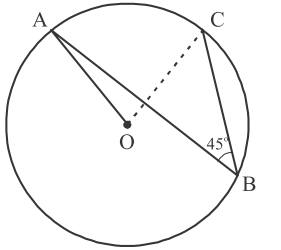Solution:

Given,

∠ABC = 45°

We know that the angle subtended by an arc of the circle at the centre is double the angle subtended at the remaining part of the circle by the same arc.

⇒ ∠AOC = 2∠ABC

= 2 × 45°

= 90°

⇒ OA ⊥ OC

Hence proved.

Question 14: O is the circumcentre of a triangle ABC and D is the midpoint of base BC. Prove that: ∠BOD = ∠A

Solution:

Given,

∆ABC with circumcenter O and D is the midpoint of BC.

∴ BD = DC

Draw OD ⊥ BC.

Join OB and OC.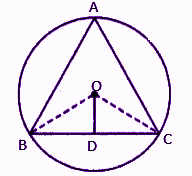In ∆OBD and ∆OCD,

OB = OC (radius of the same circle)

∠ODB = ∠ODC (each 90°)

OD = OD (common)

∴ ∆OBD ≅ ∆OCD (by SAS congruence rule)

⇒ ∠BOD = ∠COD

We know that the angle subtended by the arc BC at the center of circle i.e., ∠BOC is double the angle subtended by the same arc at A of the remaining part of the circle, i.e., ∠BAC.

⇒ ∠BOC = 2∠BOD = 2∠COD

∠BOC = 2∠A

⇒ 2∠BOD = 2∠A (since ∠BOC = 2∠BOD)

⇒ ∠BOD = ∠A

Hence proved.

Question 15: On common diagonal AB, two right triangles ACB and ADB are drawn in such a way that they are situated on opposite side, then prove that: ∠BAC = ∠BDC

Solution:

Taking AB as the diameter, draw a circle which passes through the points C and D each on the opposite side of AB.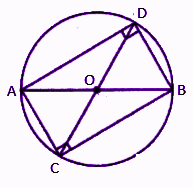∠ACB = ∠ADB = 90° (angle in semicircle is 90°)

Therefore, angles subtended by minor arc BC with the same segment will be equal.

⇒ ∠BAC = ∠BDC

Hence proved.

Question 16: Two chords AB and AC of a circle subtended angle of 90° and 150° at the centre. Find the angle BAC, if AB and AC on the opposite side of the centre.

Solution:

Given,

Two chords AB and AC of a circle subtended angle of 90° and 150° at the centre.

Join OA, OB and OC.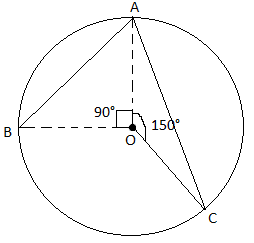∠BOA = 90° and ∠AOC = 150°

∴ ∠BOC = 360° – (∠BOA + ∠AOC)

= 360° – (90° + 150°)

= 360° – 240°

= 120°

We know that,

∠BAC = (1/2) ∠BOC = (1/2) × 120° = 60°

Therefore, ∠BAC = 60°

Question 17: If O is the circumcentre of triangle ABC, then prove that: ∠OBC + ∠BAC = 90°

Solution:

Given,

O is the circumcentre of triangle ABC.

Join OB and OC.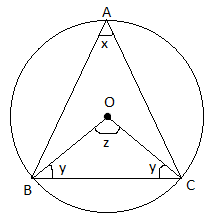We know that,

∠BOC = 2∠BAC

⇒ z = 2x ….(i)

In triangle OBC,

∠OBC + ∠OCB + ∠BOC = 180°

⇒ y + z + y = 180°

⇒ 2y + z = 180°

⇒ 2y + 2x = 180° [From (i)]

⇒ 2(x + y) = 180°

⇒ x + y = 90°

⇒ ∠BAC + ∠OBC = 90°

Hence proved.

Question 18: If the length of a chord is equal to its radius, then find out the angle on the major segment in a circle.

Solution:

Let AB be the chord which is equal to the radius.

AB = OA = OB

∆AOB is an equilateral triangle.

⇒ ∠AOB = 60°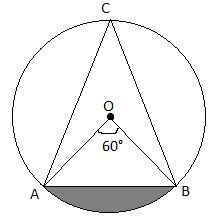Let C be any point on major segment BA.

∠ACB = (1/2)∠AOB

= (1/2) × 60°

= 30°

Hence, the required measure of angle is 30°.

Question 19: In the figure, ∠ADC = 130° and chord BC = chord BE. Find the value of ∠CBE.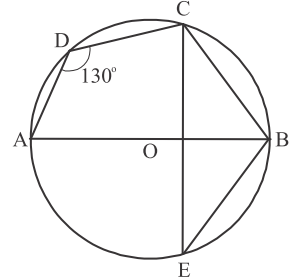Solution:

From the given figure,

We know that the sum of opposite angles of a cyclic quadrilateral is 180°.

⇒ ∠ABC = 180° – ∠ADC

⇒ ∠ABC = 180° – 130°

⇒ ∠ABC = 50°

⇒ ∠OBC = 50°

In ΔBCO and ΔBEO,

BC = BE (given)

∠BCO = ∠BEO (angles opposite to equal sides are equal)

BO = BO (common)

By SAS congruence rule,

ΔBCO ≅ ΔBEO

By CPCT,

∠OBC = ∠OBE

∴ ∠OBE = 50° (since ∠OBC = 50°)

And

∠CBE = ∠CBO + ∠OBE

= 50° + 50°

= 100°

Therefore, ∠CBE = 100°

Question 20: In the figure, ∠ACB = 40°, find the value of ∠OAB.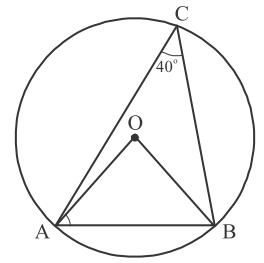Solution:

Given,

∠ACB = 40°

We know that the angle subtended by the arc of a circle at centre is double the angle at the remaining part of the circle.

∴ ∠AOB = 2∠ACB = 2 × 40° = 80°

⇒ ∠AOB = 80°

∵ OA = OB (radius of the same circle)

Angles opposite to equal sides are equal.

∴ ∠OAB = ∠OBA

In triangle AOB,

∠OAB + ∠OBA + ∠AOB = 180°

⇒ ∠OAB + ∠OAB + 80° = 180°

⇒ 2∠OAB = 180° – 80°

⇒ ∠OAB = 100°/2

⇒ ∠OAB = 50°

Question 21: In the figure, AOB is the diameter of a circle and C, D and E are three points situated on a semicircle. Find the value of ∠ACD + ∠BED.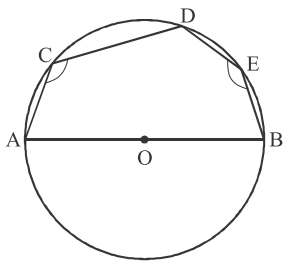Solution:

Join AE.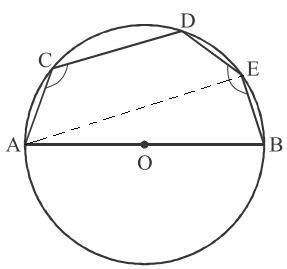∠AEB = 90° ….(i)

Opposite angles of cyclic quadrilateral are supplementary.

⇒ ∠ACD + ∠DEA = 180° ….(ii)

∠AEB + ∠DEA + ∠ACD = 180° + 90°

∠BED + ∠ACD = 270° (since ∠AEB + ∠DEA = ∠BED)

### RBSE Maths Chapter 12: Exercise 12.4 Textbook Important Questions and Solutions

Question 22: One angle of cyclic quadrilateral is given, then find the opposite angles.

(i) 70° (ii) 3/5 right angle (iii) 165°

Solution:

We know that the sum of the opposite angles of a cyclic quadrilateral is 180°.

(i) Given, one angle is 70°.

Let x be the opposite angle.

70° + x = 180°

x = 180° – 70° = 110°

(ii) (3/5) right angle = (3/5) 90° = 54°

Let x be the opposite angle.

54° + x = 180°

x = 180° – 54° = 126°

(iii) Given, one angle is 165°.

Let x be the opposite angle.

165° + x = 180°

x = 180° – 165° = 15°

Question 23: Find the opposite angle of a cyclic quadrilateral in which one angle is 2/7 th of the other angle.

Solution:

Let x be the other opposite angle.

First opposite angle = (2/7)x

We know that the sum of opposite angles of a cyclic quadrilateral is 180°.

x + (2/7)x = 180°

(7x + 2x)/7 = 180°

9x/7 = 180°

x = (180° × 7)/9

x = 140°

Therefore, the first opposite angle = (2/7)x = (2/7) × 140° = 40°

Hence, the opposite angles of the cyclic quadrilateral are 40° and 140°.

Question 24: In the figure, find all the four angles of a cyclic quadrilateral ABCD.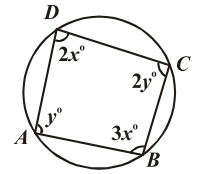Solution:

We know that the sum of opposite angles of cyclic quadrilateral is 180°

.∠A + ∠C = 180°

y + 2y = 180°

3y = 180°

y = 180°/3 = 60°

∠A = y = 60°

∠C = 2y = 2 × 60 = 120°

Similarly,

∠B + ∠D = 180°

3x + 2x = 180°

5x = 180°

x = 180°/5 = 36°

∠B = 3x = 3 × 36° = 108°

∠D = 2x = 2 × 36° = 72°

Therefore, ∠A = 60°, ∠B = 108°, ∠C = 120°, and ∠D = 72°.

Question 25: In the figure, few angles are denoted by a, b, c and d. Find the measurement of these angles.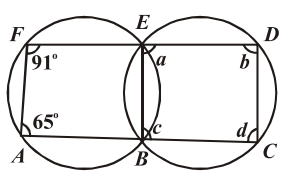Solution:

From the given figure,

ABEF and BCDE are two cyclic quadrilaterals.

We know that the sum of opposite angles of a cyclic quadrilateral is 180°.

∠FAB + ∠BEF = 180°

65° + ∠BEF = 180°

∠BEF = 180° – 65° = 115°

Similarly,

∠EBA + ∠AFE = 180°

∠EBA + 91° = 180°

∠EBA = 180° – 91° = 89°

We know that the exterior angle of a cyclic quadrilateral is equal to the opposite internal angle.

∠EBC = ∠AFE = 91°

∴ ∠EBC = c = 91°

∠DEB = ∠BAF = 65° = a = 65°

∠EBA = ∠CDE = 89°

∠CDE = b = 89°

∴ ∠BEF = ∠BCD= 115°

∴ ∠BCD = d = 115°

Therefore, ∠a = 65°, ∠b = 89°, ∠c = 91°, ∠d = 115°.

Question 26: If in a cyclic quadrilateral ABCD, AD ∥ BC, then prove that: ∠A = ∠D

Solution:

Given,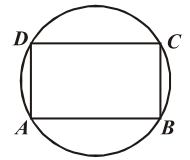We know that the sum of opposite angles of a cyclic quadrilateral is 180°.

∠A + ∠C = 180°

∠B + ∠D = 180° ….(i)

And ∠A + ∠B = 180° ….(ii) (co-interior corresponding angles)

From (i) and (ii),

⇒ 2∠A + ∠B + ∠C = ∠B + ∠C + 2∠D

⇒ 2∠A = 2∠D

⇒ ∠A = ∠D

Hence proved.

Question 27: ABCD is a cyclic quadrilateral, on increasing AB and DC, they meet each other at point E. Prove that ΔEBC and ΔEDA are similar.

Solution:

Given,

Produced AB and DC meet each other at E.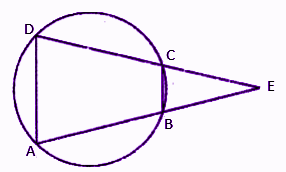We know that the exterior angle of cyclic quadrilateral is equal to the opposite interior angle.

In ∆EBC and ∆EDA,

∠EBC = ∠EDA

∠E = ∠E (common)

By AAA similarity criterion,

∆EBC ~ ∆EDA

Hence proved.

Solution:

Let ABCD be the cyclic quadrilateral.

AP, BP, CR and DR are respectively the bisectors of ∠A, ∠B, ∠C, and ∠D.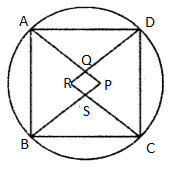We know that the sum of opposite angles of a cyclic quadrilateral is 180°.

In ∆APB and ∆CRD,

∠APB + ∠PAB + ∠PBA = 180°

∠CRD + ∠RCD + ∠RDC = 180°

∠APB + (1/2)∠A + (1/2)∠B = 180° ….(i)

And

∠CRD + (1/2)∠C + (1/2)∠D = 180° ….(ii)

∠APB + (1/2)∠A + (1/2)∠B + ∠CRD + (1/2)∠C + (1/2)∠D = 180° + 180°

∠APB + ∠CRD + (1/2)(∠A + ∠B + ∠C + ∠D) = 360°

∠APB + ∠CRD + (1/2)(180° + 180°) = 360° (opposite angles of a cyclic quadrilateral are supplementary)

∠APB + ∠CRD + (1/2)(360°) = 360°

∠APB + ∠CRD + 180° = 360°

∠APB + ∠CRD = 360° – 180°

∠APB + ∠CRD = 180°

Thus, one pair of opposite angles of quadrilateral PQRS are supplementary.

Therefore, PQRS is a cyclic quadrilateral.

Hence proved.

### RBSE Maths Chapter 12: Additional Important Questions and Solutions

Question 1: In a circle of a radius 10 cm, the length of chord which is 6 cm apart from the centre is:

(a) 16 cm

(b) 8 cm

(c) 4 cm

(d) 5 cm

Solution:

Let AB be the chord of a circle with centre O.

Radius = OA = 10 cm

Distance of the chord from the centre = OP = 6 cm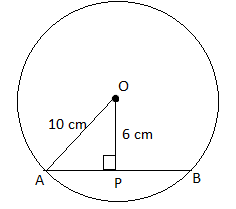In right triangle OPA,

OA2 = OP2 + AP2

AP2 = OA2 – OP2

= (10)2 – (6)2

= 100 – 36

= 64

AP = √64 = 8 cm

Chord AB = 2AP = 2(8) = 16 cm

Question 2: The measurement of short arc is:

(a) less than 180°

(b) more than 180°

(c) 360°

(d) 270°

Solution:

Diameter of a circle divides it into two equal arcs of 180°.

Hence, the measurement of the shorter arc must be less than 180°.

Question 3: The measurement of bigger arc is:

(a) less than 180°

(b) more than 180°

(c) 360°

(d) 90°

Solution:

Diameter of a circle divides it into two equal arcs of 180°.

Hence, the measurement of the bigger arc must be greater than 180°.

Question 4: In a circle, the chords are at the same distance from the centre, then one chord is ______ of the other.

(a) double

(b) triple

(c) half

(d) equal

Solution:

We know that,

In a circle, equal chords will be at equal distance from the centre.

In a circle, the chords are at the same distance from the centre, then one chord is equal to the other chord.

Question 5: If AD is a diameter of any circle and AB is chord. If AD = 34 cm, AB = 30 cm, then the distance of AB from the centre is:

(a) 17 cm

(b) 15 cm

(c) 4 cm

(d) 8 cm

Solution:

Given,

Diameter = AD = 34 cm

Chord AB = 30 cm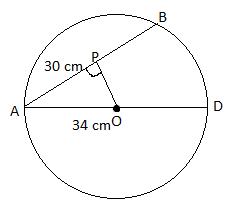OP ⊥ AP

AP = AB

AP = (1/2) × 30

AP = 15 cm

In right triangle OPA,

By Pythagoras theorem,

AO2 = OM2 + AM2

OM2 = AO2 – AM2

= 172 – 152

= 289 – 225

= 64

OP = √64

OP = 8 cm

Hence, the required distance is 8 cm.

Question 6: In the figure, if OA = 5 cm, AB = 8 cm and chord OD is perpendicular to AB, then CD is equal to: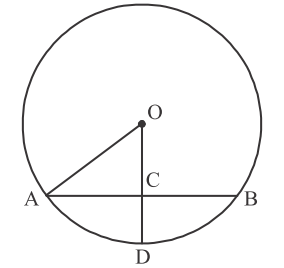(a) 2 cm

(b) 3 cm

(c) 4 cm

(d) 5 cm

Solution:

Given,

Radius = OA = 5 cm

OD ⊥ AB

∴ OC ⊥ AB

We know that the perpendicular drawn from the centre of the circle bisects the chord.

AC = BC = AB

AC = (1/2) × 8

AC = 4 cm

In right triangle OCA,

OA2 = OC2 + AC2

OC2 = OA2 – AC2

= (5)2 – (4)2

= 25 – 16 = 9

OC = √9

OC = 3 cm.

∵ OD = OA = 5 = Radius of the circle

CD = OD – OC

= 5 – 3 = 2 cm

Question 7: If AB = 12 cm, BC = 16 cm and the line segment AB is perpendicular on BC, then the radius of circle passing through A, B and C is:

(a) 6 cm

(b) 8 cm

(c) 10 cm

(d) 12 cm

Solution:

Given,

AB = 12 cm, BC = 16 cm and the line segment AB is perpendicular on BC.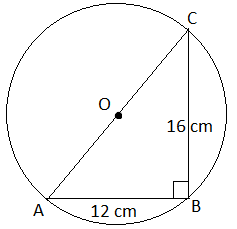We know that the angle in a semicircle is the right angle.

∴ ∠B = 90°

In right ΔABC

AC2 = AB2 + BC2

= (12)2 + (16)2

= 144 + 256

= 400

⇒ AC = √400 = 20

Diameter = 20 cm

Radius = 20/2 = 10 cm

Question 8: In the figure, if ∠ABC = 20°, then ∠AOC is equal to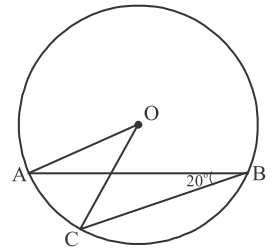(a) 20°

(b) 40°

(c) 60°

(d) 10°

Solution:

Given,

∠ABC = 20°

We know that the angle subtended by an arc at the centre of the circle is double the angle subtended at the remaining part.

⇒ ∠AOC = 2∠ABC

= 2 × 20°

= 40°

Question 9: In the figure, if AOB is a diameter of circle and AC = BC, then ∠CAB is equal to: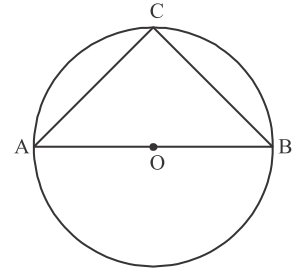(a) 30°

(b) 60°

(c) 90°

(d) 45°

Solution:

From the given figure,

∠ACB is in a semicircle.

∴ ∠ACB = 90°

AC = BC (Given)

∴ ∠CAB = ∠CBA (angles opposite to equal sides)

In triangle ACB,

∠CAB + ∠ABC + ∠BCA = 180°

= ∠CAB + ∠CAB + 90° = 180°

2∠CAB = 180 – 90°

∠CAB = 45°

Question 10: In the figure, is ∠DAB = 60° and ∠ABD = 50°, then ∠ACB is equal to: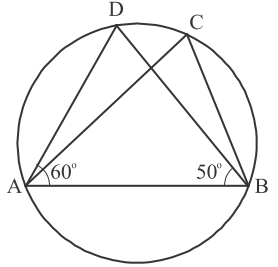(a) 60°

(b) 50°

(c) 70°

(d) 80°

Solution:

Given,

∠DAB = 60° and ∠ADB = 50°

∠DAB + ∠ABD + ∠ADB = 180°

⇒ 60° + 50° + ∠ADB = 180°

⇒ ∠ADB = 180° – (60° + 50°)

∠ACB and ∠ADB are angles in the same segment.

⇒ ∠ACB = ∠ADB = 70°

Question 11: In the figure, BC is the diameter and ∠BAO = 60°, then ∠ADC is equal to: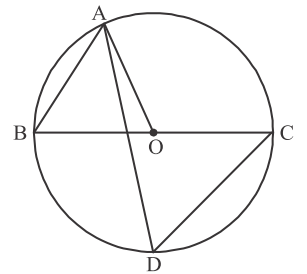(a) 30°

(b) 45°

(c) 60°

(d) 120°

Solution:

Given,

∠BAO = 60°

∴ ∠OBA = 60° (OA = OB)

⇒ ∠ADC = 60° (angles in the same segment are equal)

Question 12: In the figure, ∠AOB = 90° and ∠ABC = 30°, then ∠CAO is equal to: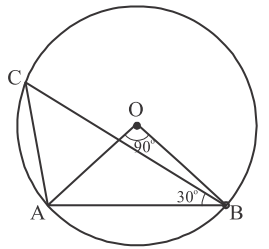(a) 30°

(b) 45°

(c) 90°

(d) 60°

Solution:

Given,

∠AOB = 90°

Radius of the circle = OA = OB

Let ∠OAB = ∠OBA = x

In ΔOAB

∠OAB + ∠OBA + ∠AOB = 180°

⇒ x + x + 90° = 180°

⇒ 2x = 180° – 90°

⇒ x = 90°/2 = 45°

∴ ∠OAB = 45°

And ∠OBA = 45°

We know that the angle subtended by arc at the centre of the circle is double the angle subtended at the remaining part of the circle.

Thus, ∠AOB = 2∠ACB

∠ACB = (1/2) ∠AOB = (1/2) × 90° = 45°

In triangle ABC,

∠ACB + ∠BAC + ∠CBA = 180°

∠ACB + (∠BAO + ∠CAO) + ∠CBA = 180°

⇒ 45° + (45° + ∠CAO) + 30° = 180°

⇒ ∠CAO = 180° – (30° + 45° + 45°)

⇒ ∠CAO = 180° – 120°

⇒ ∠CAO = 60°

Question 13: Prove that the opposite angles of cyclic quadrilateral are supplementary or sum is 180°.

Solution:

Given,

To prove: ∠A + ∠C = 180°

∠B + ∠D = 180°

Construction OB and OD.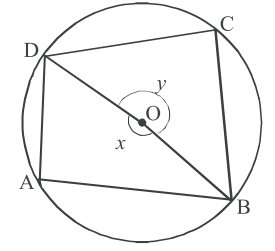Proof:

The angle made by an arc DAB at the centre of the circle is x and on the remaining part of the circle is ∠C.

Thus, ∠C = (1/2)x ….(i)

Similarly,

The angle made by an arc DCB at the centre of the circle is y and on the remaining part of the circle is ∠A.

Thus, ∠A = (1/2)y ….(ii)

∠A + ∠C = (1/2)(x + y)

∠A + ∠C = (1/2) × 360° = 180° ….(iii)

We know that, sum of all the angles of a quadrilateral = 360

∠A + ∠B + ∠C + ∠D = 360°

∠B + ∠D = 360° – (∠A + ∠C)

∠B + ∠D = 360° – 180°

∠B + ∠D = 180°

Hence proved.

Question 14: Find the ratio between the chords which are equidistant from the centre of a circle.

Solution:

We know that the equal chords of the circle are equidistant from the centre of the circle.

In vice verse, chords which are equidistant from the centre are equal.

Hence, the ratio of these chords is 1 : 1.